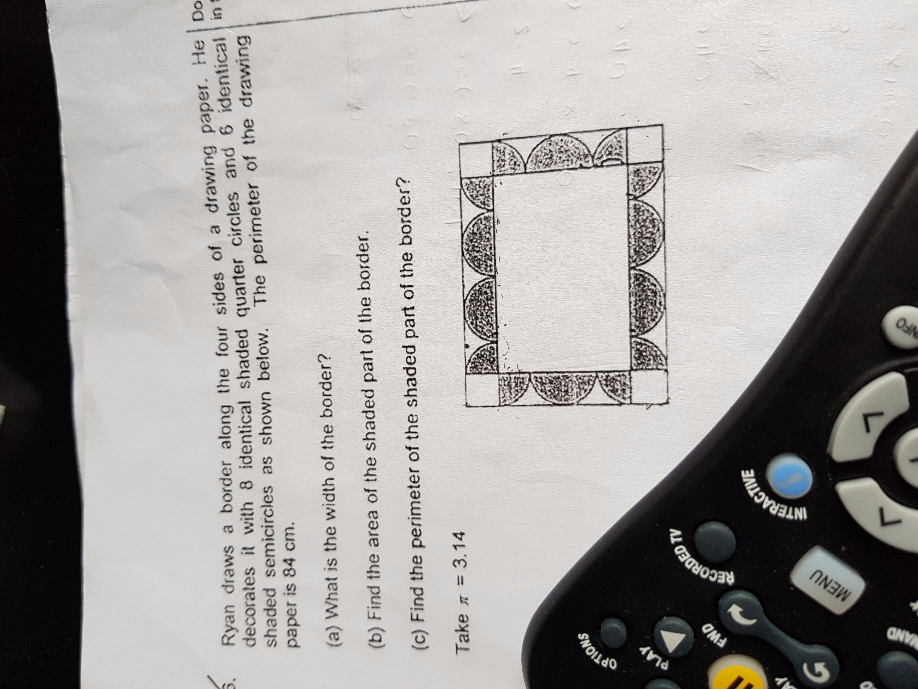# QuestionHello, any one can help with this question? Thanks

(a)
8 + 6 = 14
14 x 2 = 28
84/28 = 3
Note : The width of the border is the radius.

(b)
Area of shaded parts = area of 5 full circles ——- 5 x 3.14 x 3 x 3 = 141.3

(c)
Method 1 :
perimeter of 1 quadrant ——- [(1/4) x 2 x 3.14 x 3] + 3 + 3 = 10.71
perimeter of 20 quadrant ——- 10.71 x 20 = 214.2
214 – 3 x 6 x 2 = 178.2

Method 2 :
5 x 2 x 3.14 x 3 = 94.2
(20 + 8) x 3 = 84
84 + 94.2 = 178.2

Ans : (a) 3cm; (b) 141.3 sq cm; (c) 178.2cm.

0 Replies 1 Like
0 Replies 0 Likes